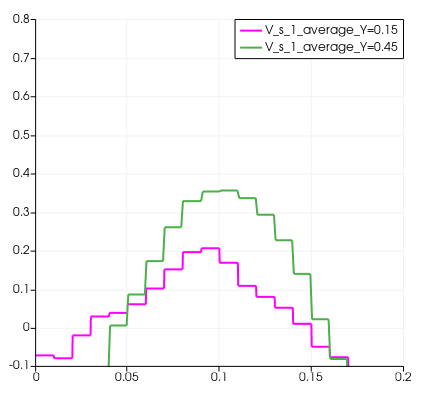# How to get smooth line plot in paraview

I am trying to make plot velocity vs length-wise, but the plot data is coming in the form of steps. Can you please tell me how can I get a smooth line plot?Are you making this plot with `Plot Over Line`? If so, it looks like you are doing it with cell-centered data. Try running the `Cell Data to Point Data` filter before `Plot Over Line`.

I plot the Temporalstatics point data to an over-the-line plot. but the same plot is coming as shown below.
then how can I get a smooth plot without any curve fitting?